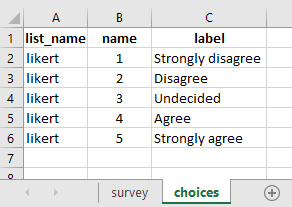# Reverse score calculations (composite index)

Hi there.

I have an 18 question tool with a 5 point likert response scale (1 = Strongly disagree 2 = Disagree 3 = Undecided 4 = Agree 5 = Strongly agree).

I would like to calculate a total score for each respondent but have 8 questions where these need to be reverse scored. So items 1, 2, 5, 6, 7, 8, 17, and 18 should be reverse scored as follows: (1=5) (2=4) (3=3) (4=2) (5=1).

I have tried this for the reverse scored questions
if(\${Q1}=‘Strongly disagree’,5,0)+if(\${Q1}=‘Disagree’,4,0)+if(\${Q1}=‘Undecided’,3,0)+if(\${Q1}=‘Agree’,2,0)+if(\${Q1}=‘Strongly agree’,1,0)

And this for the others
if(\${Q3}=‘Strongly disagree’,1,0)+if(\${Q1}=‘Disagree’,2,0)+if(\${Q1}=‘Undecided’,3,0)+if(\${Q1}=‘Agree’,4,0)+if(\${Q1}=‘Strongly agree’,5,0)

But I keep getting an error message that the “parser is broken”

I would appreciate some help with the expressions for the calculation.

Many thanks,

Alice

Welcome to the community @ashirley! Maybe you could do the same as outlined in the image as shared below:

### In the choices tab of your xlsform:### Reference xlsform:

Weighted Score Sample.xlsx (11.7 KB)

1 Like

Thank you! That has worked. I just reversed the score calculation for the relevant questions.

2 Likes